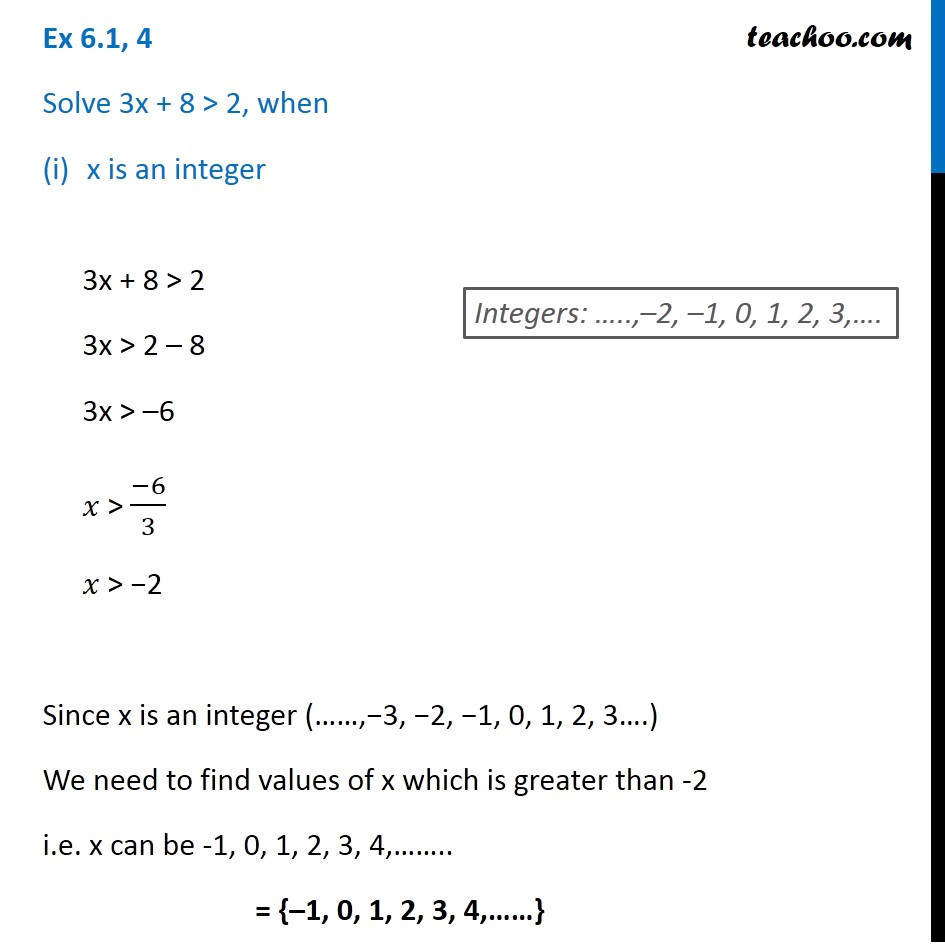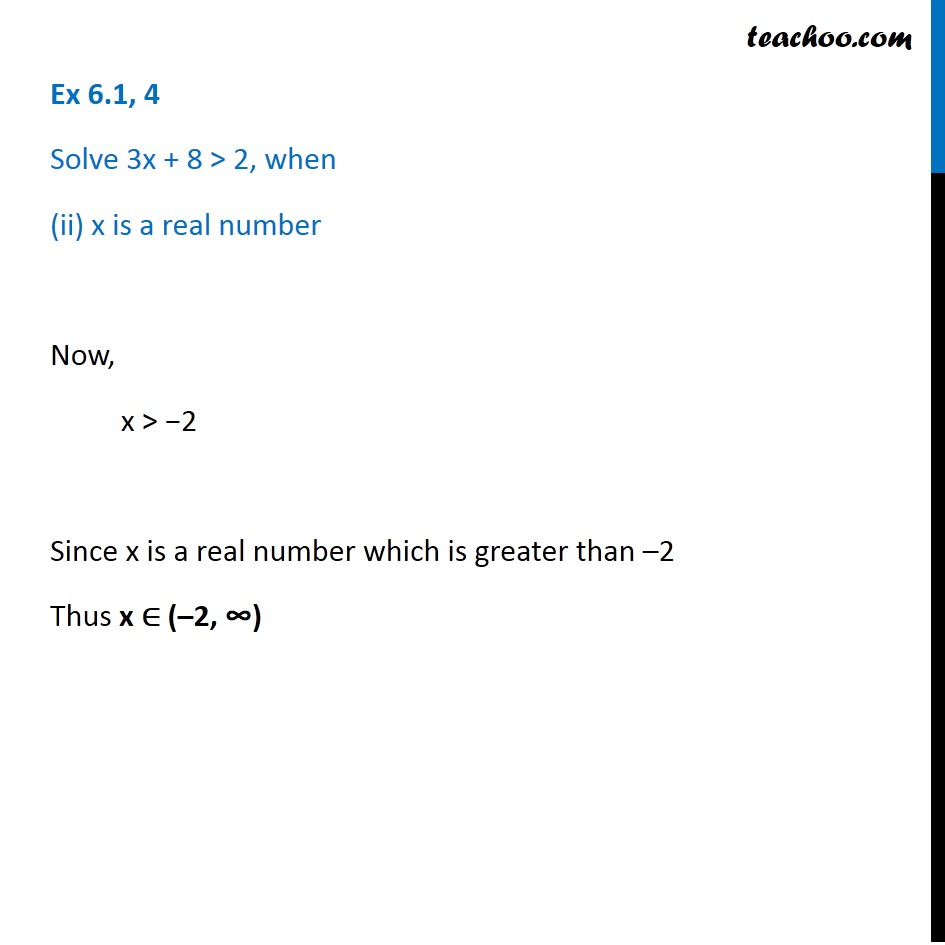1. Chapter 6 Class 11 Linear Inequalities (Term 2)
2. Serial order wise
3. Ex 6.1

Transcript

Ex 6.1, 4 Solve 3x + 8 > 2, when x is an integer 3x + 8 > 2 3x > 2 – 8 3x > –6 𝑥 > (−6)/3 𝑥 > −2 Since x is an integer (……,−3, −2, −1, 0, 1, 2, 3….) We need to find values of x which is greater than -2 i.e. x can be -1, 0, 1, 2, 3, 4,…….. = {–1, 0, 1, 2, 3, 4,……} Integers: …..,–2, –1, 0, 1, 2, 3,…. Ex 6.1, 4 Solve 3x + 8 > 2, when (ii) x is a real number Now, x > −2 Since x is a real number which is greater than –2 Thus x ∈ (–2, ∞)